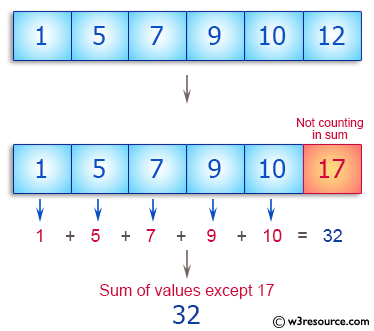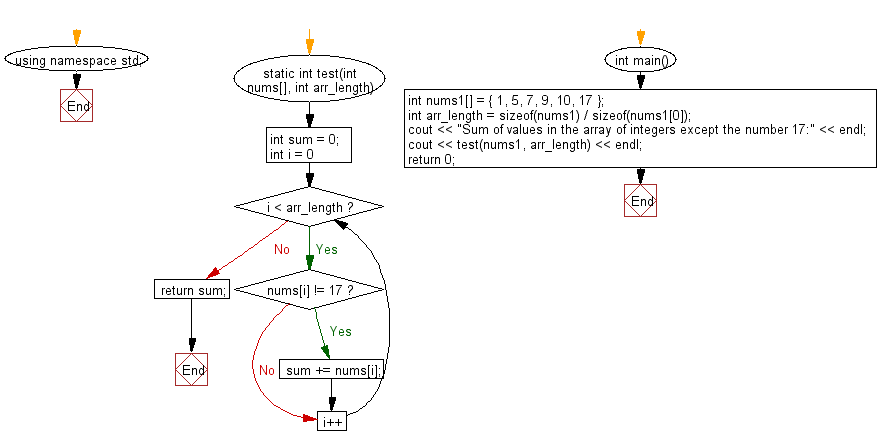﻿ C++ : Compute the sum of values in an array except 17# C++ Exercises: Compute the sum of values in a given array of integers except the number 17

## C++ Basic Algorithm: Exercise-100 with Solution

Write a C++ program to compute the sum of values in a given array of integers except the number 17. Return 0 if the given array has no integer.

Sample Solution:

C++ Code :

``````#include <iostream>
using namespace std;

static int test(int nums[], int arr_length)
{
int sum = 0;

for (int i = 0; i < arr_length; i++)
{
if (nums[i] != 17) sum += nums[i];
}
return sum;
}

int main()
{
int nums1[] = { 1, 5, 7, 9, 10, 17 };
int arr_length = sizeof(nums1) / sizeof(nums1);
cout << "Sum of values in the array of integers except the number 17:" << endl;
cout << test(nums1, arr_length) << endl;
return 0;
}
``````

Sample Output:

```Sum of values in the array of integers except the number 17:
32
```

Pictorial Presentation:Flowchart:C++ Code Editor: